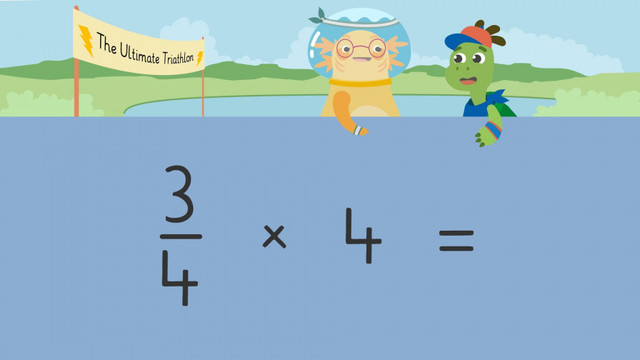# Multiplying a Fraction by a Whole NumberRate this video

Ø 5.0 / 1 ratings

The authorTeam Digital
Multiplying a Fraction by a Whole Number
CCSS.MATH.CONTENT.4.NF.B.4.B

## Information about the videoMultiplying a Fraction by a Whole Number

### Contents

#### How to multiply fractions with whole numbers Steps to multiplying a whole number by a fraction: First, write the fraction Next, write the whole number as a fraction with a denominator of one. Multiply the numerators. Multiply the denominators. Rewrite the fraction as a mixed number if needed.

#### In this video

Axel and Tank decide to take part in The Ultimate Triathlon. They are going to need to spend a portion of time each week on biking, swimming, and running in order to prepare for the event. We can help Axel and Tank get ready for the event by "Multiplying Fractions by Whole Numbers" to keep track of their training routines.

This video will show multiplying a fraction by a whole number using models and multiplying a fraction by a whole number using symbols,

#### Examples of multiplying a fraction by a whole numberFor continued practice, there is a multiplying a fraction by a whole number worksheet available.

### TranscriptMultiplying a Fraction by a Whole Number

“....So, start training and come on down to participate in the Ultimate Triathlon!” “Tank,(...) are you thinking what I’m thinking?” “That it’s time for dinner?” “ No (...) well, yes (...) but, I think we should take part in the triathlon!” Axel and Tank are going to need to spend a portion of time each week on biking, swimming, and running in order to prepare for the triathlon. We can help Axel and Tank get ready for the event by "Multiplying Fractions by Whole Numbers" to keep track of their training routines. Axel and Tank are going to bike for two-thirds of an hour, (...)twice a week. We know that we can add fractions to solve for the total. Two-thirds plus two-thirds equals four-thirds. Although, repeated addition becomes tricky when we have more groups. We can also multiply fractions by whole numbers because it is a more efficient way to solve the problem. To multiply fractions by a whole number, first, start with the fraction that is shown(...) two-thirds. We are going to multiply that by the number of groups we have(...) which is two. Next, rewrite the whole number as a fraction. So we need to add a denominator. Since a denominator tells us how many parts a whole is divided into, think about how many parts make up a whole. A whole number is NOT divided into parts, so there is only 1 in the WHOLE... meaning the denominator is ONE. Now, let's multiply two-thirds times two. (...) Multiply the numerators… two times two equals four. Now multiply the denominators… three times one equals three. Two-thirds times two equals four-thirds. Four-thirds is an improper fraction because four is more than three, so it can be rewritten as one and one-third. Axel and Tank will ride their bikes for one and one-third hours each week. They will also need to train for the running event. To set up the multiplication equation for this problem, the first step is to write the fraction. What is the fraction of the hour they plan on training? (...) They will train for three-fourths of an hour. How many times a week will they run? They will run four times a week. Our equation is three-fourths times four. The next step is to rewrite four as a fraction by putting one as the denominator. Now, multiply the numerators(...) three times four equals twelve… and multiply the denominators(...) four times one equals four. The answer is twelve-fourths(...) which can be rewritten as three wholes. Axel and Tank will run three hours a week. Since swimming is their favorite, they are planning on swimming A LOT! How much time in an hour do they plan to spend on swimming? They are going to swim eight-twelfths of an hour. How many times a week are they going to swim? Nine times a week. How do we set up the equation? Eight-twelfths times nine over one equals. How many hours a week do they train for swimming? Seventy-two twelves(...) which can be rewritten as six. Axel and Tank will swim six hours each week!! Remember,(...) We multiply fractions by whole numbers because it is a more efficient way to solve the problem. First, write the fraction. Next, write the whole number as a fraction with a denominator of one. Multiply the numerators. Multiply the denominators Rewrite the fraction as a mixed number if needed. “TANK, (...) WE DID IT!” “Yes! All that hard work paid off!” “It was a beautiful day today at The Ultimate Triathlon…” we are SO proud of all the contestants.”